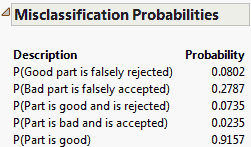Publication date: 05/24/2021

## Misclassification Probabilities

Due to measurement variation, good parts can be rejected and bad parts can be accepted. This is called misclassification. Misclassification rates decrease as measurement variability decreases. When you select the Misclassification Probabilities option, you are prompted to select the model type and enter specification limits if you have not already done so.

Figure 5.8 Example of the Misclassification Probabilities ReportThe misclassification probabilities are based on the joint probability function of Y, the measured value of the part, and X, the true value of the part. The joint probability density function used is a bivariate normal distribution. To understand the descriptions, define the following probabilities:

δ = P[(LSL X USL) and (Y < LSL or Y > USL)]

β = P[(X < LSL or X > USL) and (LSL Y USL)]

π = P(LSL X USL)

#### Descriptions

P(Good part is falsely rejected)

The conditional probability that a part is rejected given that it is a good part, or δ/π.

The conditional probability that a part is accepted given that it is a bad part, or β/(1-π).

P(Part is good and is rejected)

The joint probability that a part is good and that it is rejected, or δ.

P(Part is bad and is accepted)

The joint probability that a part is bad and that it is accepted, or β.

P(Part is good)

The probability that a part is good, or π.

For more information, see Statistical Details for the Misclassification Probabilities as well as Burdick et al. (2005).